×#### Thank you for registering.

One of our academic counsellors will contact you within 1 working day.

Click to Chat

1800-1023-196

+91-120-4616500

CART 0

• 0

MY CART (5)

Use Coupon: CART20 and get 20% off on all online Study Material

ITEM
DETAILS
MRP
DISCOUNT
FINAL PRICE
Total Price: Rs.

There are no items in this cart.
Continue Shopping• Complete JEE Main/Advanced Course and Test Series
• OFFERED PRICE: Rs. 15,900
• View Details

```Chapter 15: Pair of Lines and Transversal Exercise 15.1

Question: 1

Identify parallel line segments: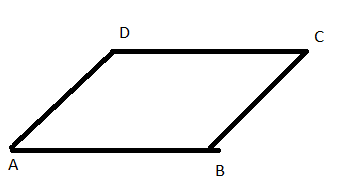(i)
(ii)
(iii)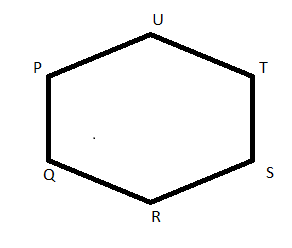(iv)
(v)
(vi)

Solution:

(i) BC ǁ DE

(ii) AB ǁ DC, AD ǁ BC

(iii) AB ǁ DC, AD ǁ BC

(iv) PQ ǁ TS, UT ǁ QR , UP ǁ SR

(v) AB ǁ DC ǁ EF, AD ǁ BC and DE ǁ CF

(vi) BC ǁ E, AB ǁ DF and AC ǁ DE

Question: 2

Name the pairs of all possible parallel edges of the pencil box whose figure is shown in the figureSolution:

(i) AH ǁ DG ǁ CF ǁ BE

(ii) AB ǁ DC ǁ GF ǁ HE

(iii) AD ǁ HG ǁ EF ǁ BC

Question: 3

In the figure, do the segments AB and CD intersect? Are they parallel? Give reasons.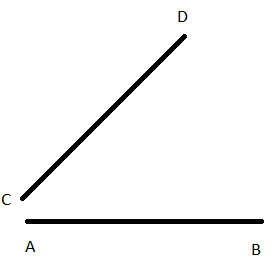Solution:

In the given position, segments AB and CD do not intersect, but hey can if extended to a point. No, they are not parallel, as the distance between them is not constant.

Question: 4

State which of the following are true or false:

i) If two lines in the same plane do not intersect, then they must be parallel

ii) Distance between two parallel lines is not same everywhere

iii) If m perpendicular l and n perpendicular l and m ≠ n, then m parallel to n

iv) Two non – intersecting co –planar rays are parallel

iv) If Ray AB parallel to m, then line segment AB parallel to m

v) If Ray AB parallel to m, then line segment AB parallel to m

vi) No two parallel segments intersect each other

vii) Every pair of lines is a pair of co-planar lines

viii) Two lines perpendicular to the same line are parallel

ix) A line perpendicular to one of two parallel lines is perpendicular to each other

Solution:

State which of the following are true or false:

i) True

ii) False

iii) True

iv) False

iv) True

v) True

vi) True

vii) False

viii) True

ix) True

Question: 5

i) Alternate corresponding angles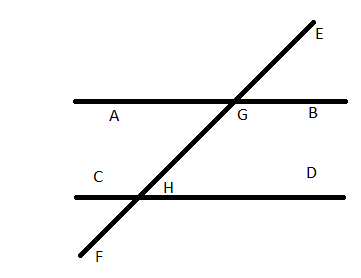ii) Angles alternate to ∠d and ∠g and angles corresponding to angles ∠f and ∠h in the figure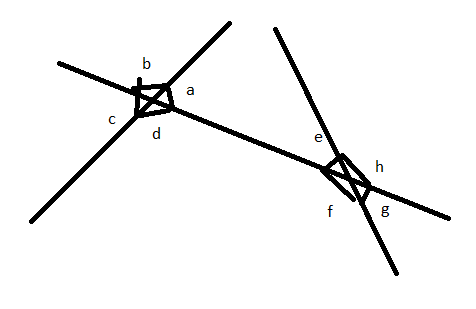iii) Angles alternative to ∠PQR, angle corresponding to ∠RQF and angle alternative to ∠PQE in the figure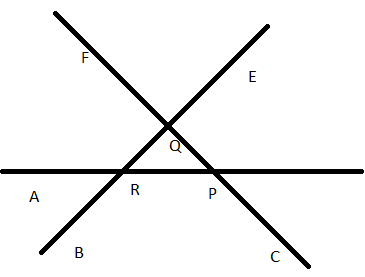Solution:

i)

Alternate interior angles are:

Angle BGH and angle CHG

Angle AGH and angle CHF

Alternate exterior angles:

Angle AGE and angle DHF

Angle EGB and angle CHF

Corresponding angles are:

Angle EGB and angle GHD

Angle EGA and angle GHC

Angle BGH and angle DHF

Angle AGF and angle CHF

ii)

The alternate angle to ∠d is ∠e and alternate angles to ∠g is ∠b

The corresponding angles to ∠f is ∠c and ∠h is ∠a

iii)

In the given figure. ‘I’ is a transversal to ‘m’ and ‘n’

So, the alternate angle of ∠PQR is ∠QRA

The corresponding angle ∠RQF and ∠BRA

The alternate angle of ∠PQE is ∠BRA

Question: 6

Match column A and column B.

i) Vertically opposite angles → a. ∠PAB and ∠ABS

ii) Alternate angles → b – ∠PAB and ∠RBY

iii) Corresponding angles → c. ∠PAB and ∠XAQ

Solution:

i) Vertically opposite angles → c. ∠PAB and ∠XAQ

ii) Alternate angles →  a. ∠PAB and ∠ABS

iii) Corresponding angles → b – ∠PAB and ∠RBY
```### Course Features

• 728 Video Lectures
• Revision Notes
• Previous Year Papers
• Mind Map
• Study Planner
• NCERT Solutions
• Discussion Forum
• Test paper with Video Solution